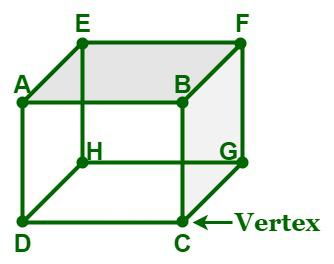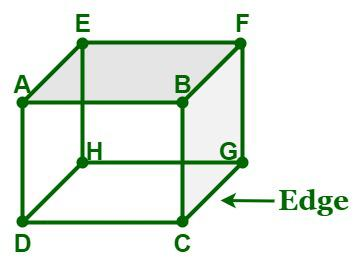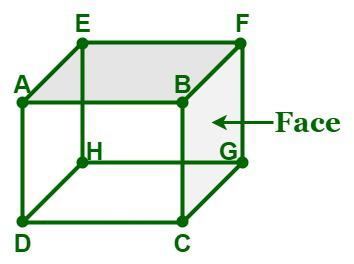GFG App
Open AppBrowser
Continue

# Vertices, Faces, and Edges

Vertices, Faces, and Edges are the three basic properties that are used to define various 3-D objects. We come across a variety of objects in our daily lives that are of different shapes and sizes. They have different dimensions like length, width, and height. For example, a rectangular field has two dimensions, i.e., length and breadth, whereas a cuboidal container has three dimensions, i.e., length, breadth, and height. Apart from the three dimensions, a 3D object is made up of different parts like vertices, edges, and faces. A solid 3D object that is made up of flat polygonal faces, straight edges, and sharp corners called vertices is called a polyhedron. In this article, we will discuss vertices, faces, and edges in detail.

## What are Vertices?

In geometry, a vertex is a point of intersection of two or more curves, lines, or edges. They are represented as points and denoted by letters such as A, B, C, D, etc. The figure given below is a cuboid that has eight vertices, i.e., A, B, C, D, E, F, G, and H.## What are Edges?

An edge is defined as the line segment where the faces of a solid meet. Even though many shapes have straight edges and lines, some shapes, like hemispheres, have curved edges. The figure given below is a cuboid that has twelve edges, i.e., AB, BC, CD, AD, DH, EH, AE, HG, CG, GF, EF, and BF.## What are Faces?

A face is defined as the flat surface of a solid. It can be referred to as the outer surface of a solid object, whether it be a curved face or a straight face. The figure given below is a cuboid that has six faces, i.e., ABCD, EFGH, ADHE, AEFB, BFGC, and DHGC.## What is a Polyhedron?

In geometry, a polyhedron is referred to as a three-dimensional solid that is made up of polygons. A polyhedron consists of flat polygonal faces, straight edges, and sharp corners called vertices. Some examples of polyhedrons are cubes, pyramids, prisms, etc. As cones, cylinders, and spheres do not have polygonal faces and curved surfaces, they are non-polyhedrons. We have different types of polyhedrons, such as regular polyhedrons, irregular polyhedrons, convex polyhedrons, and concave polyhedrons.

• Regular polyhedron: A regular polyhedron, or platonic solid, is a polyhedron whose faces are regular polygons and are congruent to each other. Tetrahedrons, cubes, regular octahedrons, regular dodecahedrons, and regular icosahedrons are some examples of regular polyhedrons.
• Irregular polyhedron: An irregular polyhedron is made up of irregular polygonal faces that are not congruent to each other. Pentagonal pyramids, triangular prisms, octagonal prisms, etc. are some examples of irregular polyhedrons.
• Convex polyhedron: A polyhedron is said to be a convex polyhedron if the surface of the polyhedron (which consists of its faces, edges, and vertices) does not intersect itself and the line segment joining any two points of the polyhedron lies within its interior part or surface.
• Concave polyhedron: A polyhedron is said to be a concave polyhedron if it is a non-convex polyhedron.

## Euler’s Formula

The relationship between vertices, faces, and edges can be determined using Euler’s formula. Euler’s formula states that for any convex polyhedron, the sum of the number of faces (F) and vertices (V) is exactly two greater than the number of edges (E). Remember that Euler’s formula is applied to closed solids that have flat sides and straight edges, such as cuboids. It is not applied to solids that have curved edges, such as cylinders, spheres, etc.

### Euler’s Formula

F + V = 2 + E

where,
F is the number of faces,
V is the number of vertices,
E is the number of edges

## Vertices, Faces, and Edges of some common 3D shapes

Now we will find the vertices, faces and edges of various solid shapes with the help of a table given below.

## Solved Examples on Euler’s Formula

Example 1: Verify Euler’s formula for the heptahedron.

Solution:

A heptahedron has 7 faces, 10 vertices, and 15 edges.

From Euler’s formula, we have,

F + V = 2 + E

⇒ 7 + 10 = 2 + 15

⇒ 17 = 17

Hence, Euler’s formula is verified for the heptahedron.

Example 2: Calculate the number of edges of a polyhedron if it has 6 vertices and 9 faces.

Solution:

Given data,

V = 6 and F = 5

From Euler’s formula, we have,

F + V = 2 + E

⇒ 5 + 6 = 2 + E

⇒ 11 = 2 + E

⇒ E = 11 – 2 = 9

Hence, the given polyhedron has 9 edges.

Example 3: Calculate the number of vertices of a polyhedron if it has 5 faces and 8 edges.

Solution:

Given data,

F = 5 and E = 8

From Euler’s formula, we have,

F + V = 2 + E

⇒ 5 + V = 2 + 8

⇒ 5 + V = 10

⇒ V = 10 – 5 = 5

Hence, the given polyhedron has 5 vertices.

Example 4: Can we have a polyhedron with 8 faces, 14 vertices, and 16 edges?

Solution:

Given data,

F = 8, V = 14, and E = 16

From Euler’s formula, we have,

F + V = 2 + E

⇒ 8 + 14 = 16 + 2

⇒ 22 ≠ 18

So, we cannot have a polyhedron with 8 faces, 14 vertices, and 16 edges.

Example 5: Calculate the number of faces of a polyhedron if it has 12 vertices and 18 edges.

Solution:

Given data,

V = 12 and E = 18

From Euler’s formula, we have,

F + V = 2 + E

⇒ F + 12 = 2 + 18

⇒ F + 12 = 20

⇒ F = 20 – 12 = 8

Hence, the given polyhedron has 8 faces.

## FAQs on Vertices, Faces, and Edges

Question 1: What is a polyhedron?

In geometry, a polyhedron is referred to as a three-dimensional solid that is made up of polygons. A polyhedron consists of flat polygonal faces, straight edges, and sharp corners called vertices. Some examples of polyhedrons are cubes, pyramids, prisms, etc.

Question 2: What is a vertex?

In geometry, a vertex is a point of intersection of two or more curves, lines, or edges. For example, a cube has eight vertices, a tetrahedron has four vertices, a cone has one vertex, whereas a cylinder and a sphere have no vertices.

Question 3: What is a face of a solid?

A face is defined as the flat surface of a solid. It can be referred to as the outer surface of a solid object, whether it be a curved face or a straight face. For example, a cube has six faces, a tetrahedron has four faces, a cylinder has three faces, i.e., a curved face and two circular faces, a cone has two faces, i.e., a curved face and a flat face, whereas a sphere has a curved surface.

Question 4: State Euler’s formula.

The relationship between vertices, faces, and edges can be determined using Euler’s formula. Euler’s formula states that for any convex polyhedron, the sum of the number of faces (F) and vertices (V) is exactly two greater than the number of edges (E).

Euler’s Formula:

F + V = 2 + E

where,
F is the number of faces,
V is the number of vertices,
E is the number of edges

### Related Resources

My Personal Notes arrow_drop_up